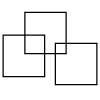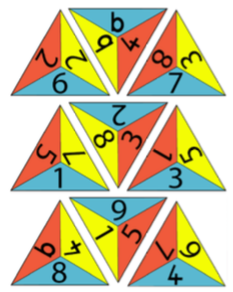#### You may also like### Halving

These pictures show squares split into halves. Can you find other ways?### Three Squares

What is the greatest number of squares you can make by overlapping three squares?### Two Dice

Find all the numbers that can be made by adding the dots on two dice.

# One Big Triangle

##### Age 5 to 7 Challenge Level:

Here are nine triangles.  Each one has three numbers on it.Your challenge is to arrange these triangles to make one big triangle, so the numbers that touch add up to 10.

You can print out this copy of the triangles and cut them out, then try arranging them.
If you prefer, here is a printable version of the triangles with the numbers represented on tens frames.

Let us know how you go about trying to make the big triangle.
How did you get started?
What did you do next?